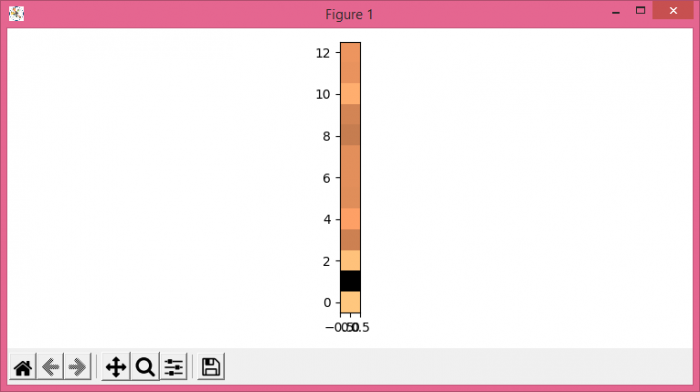# How to plot MFCC in Python using Matplotlib?

To plot MFCC in Python, we can take the following steps −

• Set the figure size and adjust the padding between and around the subplots.
• Open and read a WAV file.
• Compute MFCC features from an audio signal.
• Create a figure and a set of subplots.
• Interchange two axes of an array
• Display the data as an image, i.e., on a 2D regular raster.
• To display the figure, use show() method.

## Example

from python_speech_features import mfcc
import scipy.io.wavfile as wav
import matplotlib.pyplot as plt
import numpy as np

plt.rcParams["figure.figsize"] = [7.50, 3.50]
plt.rcParams["figure.autolayout"] = True

mfcc_data = mfcc(sig, rate)
fig, ax = plt.subplots()
mfcc_data = np.swapaxes(mfcc_data, 0, 1)

cax = ax.imshow(mfcc_data, interpolation='nearest', cmap='copper', origin='lower')

plt.show()

## Output